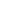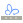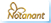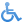Simple but complete full-profile conjoint analysisMany people ask how the elements of conjoint analysis relate to each other - how do you assign attributes and levels, build profiles and get to a calculation of part-worths or utility scores. In can be easy to talk about conjoint analysis in abstract without quite getting the practical 'this is how it works' element.

One of the original flavours or types of conjoint analysis is Full Profile and this is relatively simple to demonstrate. The attached Excel spreadsheet 📎 shows how a simple small full-profile conjoint analysis design can be built and analysed using Excel.

Conjoint analysis illustration - creating the profiles

Once the conjoint approach has been chosen, there are four basic elements of designing conjoint research to work through. Firstly, to take the attributes and levels and create a set of profiles to test on respondents. Here initially we're looking at attributes of a bicycle with four two level attributes (you can change the text to different levels for different products). Because of the simplicity of this design (only 4^2=16 options for a complete profile set) it isn't necessary to use a fractional factorial design (that is a design where not all possible combinations are used, but profiles are specially selected to maximise the data that can be collected while minimising the number of profiles to be used). In this case all combinations can be used, although this does entail some repetition for the respondent.

Specifying the profiles

In the Excel sheet there are are four two level attributes The text for the levels can be freely changed without affecting the conjoint design and this will be directly reflected in the profiles generated in the section below the attribute text. Beneath this is the 'dummy variable coding' used to determine which level is shown in each profile. There are 16 lines for the 16 combinations. These dummy variables are also used in the analysis at the end.

The generated profiles

Beneath this are the generated profiles. A simple Excel lookup function to combine the coding with the text to produce the profiles. In a normal conjoint study, you would print these full profiles on to cards and then ask respondents to rank or rate each profile according to it's acceptability.

Note that other forms of conjoint analysis use different tasks for the respondent. In Adaptive Conjoint Analysis (ACA), two or three levels are shown in a pairwise fashion (A v B) and the respondent asked to show their level of preference between the two. This is similar to the approach used in our conjoint analysis demonstration.

Alternatively in choice-based conjoint analysis (CBC or choice analysis), the profiles are used to create a choice task where the respondent chooses their preferred profile, or none if none are acceptable. This is considered closer to a real purchasing decision than the somewhat artificial ranking or rating, although it means less preference data is available for analysis and so data has to be aggregated across a sample, rather than at the individual level.

Profile ranking or rating

Having had the respondent rate or rank the data in an appropriate market research interview, the ranking or rating date is then entered into the Excel sheet in the blue area marked as Ranking. Because the ranks run 1=best to 16=worst, it is sometimes the rank score may be reversed (so the best is 16 and the worst is 1) so that in analysis, more preferred attributes will have higher utility scores. The sheet will then automatically do the calculations using Excel's linear regression LINEST function.

Calculations

From the ranks and knowing which levels apply to which profile it is possible to do a 'dummy variable regression' which relates the presence of each level as dependent variables (x's) against the independent variable (y's) of the rank given each profile. In Excel this can be calculated using the LINEST array formula - just be careful, the results are in reverse order compared to the order supplied so they need to be reversed so betas are correctly related to each level.

To make the demonstration easier to follow, we actually introduction a technical error - strictly speaking, in the version shown there is redundancy in the dummy variable coding - you only need to code for the presence or absence of one level - logically where level one is not present, level two is. This redundancy can be removed by the reader, and it may at times mean that the linear regression calculations break down.

Utilities

The results of the analysis are calculated as a set of betas and a constant in the utility line (read up on the LINEST function in Excel's help for more assistance in understanding what linear regression is trying to do). For conjoint analysis, the betas are treated as the output 'utilities' or 'part-worths' but may be scaled into more useful units for reporting as we have here. Utilities are 'unitless' so you can rescale without losing accuracy. However, each set of utilities is unique to the study in question - you cannot compare utilities across studies.

Modelling

In a full profile design, the calculated utility data is available for each respondent (this is not true for choice-based conjoint). The question arises as to which product design would be most popular in the market, or more subtly, which would be the most profitable. By treating all the respondents in your sample as representing the market as a whole, as this conjoint modelling demonstration shows, modelling works out which product would be preferred by each individual respondent based on their individual utility scores. By counting the number of respondents who would choose each product it becomes possible to estimate potential market share in a competitive market situation (called Share of Preference as it does not include aspects such as distribution or promotional effects).